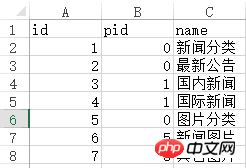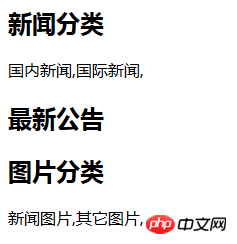# 详解PHP中foreach的用法和实例

foreach有两种语法：

`foreach (array_expression as \$value)登录后复制`

`foreach (array_expression as \$key => \$value)登录后复制`

`\$a = array('Tom','Mary','Peter','Jack');登录后复制`

1、我们用第一种foreach方法来输出。

```foreach (\$a as \$value) { echo \$value."<br/>"; }登录后复制```

```Tom Mary Peter Jack登录后复制登录后复制```

2、我们用第二种 foreach 方法来输出。

```foreach (\$a as \$key => \$value) { echo \$key.','.\$value."<br/>"; }登录后复制```

```0,Tom 1,Mary 2,Peter 3,Jack登录后复制```

1.PHP foreach的两种用法 as \$key => \$value
2.深入理解foreach语句循环数组的用法

1.独孤九贱(4)_PHP视频教程

`\$b = array('a'=>'Tom','b'=>'Mary','c'=>'Peter','d'=>'Jack');登录后复制`

```\$b = array( 'a'=>'Tom', 'b'=>'Mary', 'c'=>'Peter', 'd'=>'Jack' );登录后复制```

1、我们用第一种foreach方法来输出和上边一样。

```foreach (\$b as \$value) { echo \$value."<br/>"; }登录后复制```

```Tom Mary Peter Jack登录后复制登录后复制```

2、我们用第二种foreach方法来输出。

```foreach (\$b as \$key => \$value) { echo \$key.','.\$value."<br/>"; }登录后复制```

```a,Tom b,Mary c,Peter d,Jack登录后复制```

```\$c = array( array('1','Tom'), array('2','Mary'), array('3','Peter'), array('4','Jack') );登录后复制```

1、我们采用第一种 foreach方法：

```foreach (\$c as \$value) { print_r(\$value); echo "<br/>"; }登录后复制```

```Array (  => 11  => Tom ) Array (  => 22  => Mary ) Array (  => 33  => Peter ) Array (  => 44  => Jack )登录后复制```

2、我们采用第二种foreach方法：

```foreach (\$c as \$key => \$value) { echo '\$key='.\$key."<br/>"; print_r(\$value); echo "<br/>"; }登录后复制```

```\$key=0 Array (  => 11  => Tom ) \$key=1 Array (  => 22  => Mary ) \$key=2 Array (  => 33  => Peter ) \$key=3 Array (  => 44  => Jack )登录后复制```

```\$d = array( array('id'=>'11','name'=>'Tom'), array('id'=>'22','name'=>'Mary'), array('id'=>'33','name'=>'Peter'), array('id'=>'44','name'=>'Jack') );登录后复制登录后复制```

1、用第一种方法代码：

```foreach (\$d as \$value) { print_r(\$value); echo "<br/>"; }登录后复制```

```Array ( [id] => 11 [name] => Tom ) Array ( [id] => 22 [name] => Mary ) Array ( [id] => 33 [name] => Peter ) Array ( [id] => 44 [name] => Jack )登录后复制```

2、用第二种方法的代码：

```foreach (\$d as \$key => \$value) { echo '\$key='.\$key."<br/>"; print_r(\$value); echo "<br/>"; }登录后复制```

```\$key=0 Array ( [id] => 11 [name] => Tom ) \$key=1 Array ( [id] => 22 [name] => Mary ) \$key=2 Array ( [id] => 33 [name] => Peter ) \$key=3 Array ( [id] => 44 [name] => Jack )登录后复制```

```\$d = array( array('id'=>'11','name'=>'Tom'), array('id'=>'22','name'=>'Mary'), array('id'=>'33','name'=>'Peter'), array('id'=>'44','name'=>'Jack') );登录后复制登录后复制```

```foreach (\$d as \$key => \$value) { echo (\$value['name']); echo "<br/>"; }登录后复制```

```//获取name列作为一维数组 \$nameArr = array(); //name列 foreach (\$d as \$key => \$value) { \$nameArr[] = \$value['name']; } print_r(\$nameArr);登录后复制```

```Array (  => Tom  => Mary  => Peter  => Jack )登录后复制```

`\$d = array('Tom','Mary','Peter','Jack');登录后复制`

```//从数据库中取出的分类数据 \$original_array = array( array('id' => 1,'pid' => 0,'name' => '新闻分类'), array('id' => 2,'pid' => 0,'name' => '最新公告'), array('id' => 3,'pid' => 1,'name' => '国内新闻'), array('id' => 4,'pid' => 1,'name' => '国际新闻'), array('id' => 5,'pid' => 0,'name' => '图片分类'), array('id' => 6,'pid' => 5,'name' => '新闻图片'), array('id' => 7,'pid' => 5,'name' => '其它图片') );登录后复制``````//从数据库中取出的分类数据 \$original_array = array( array( 'id' => 1, 'pid' => 0, 'name' => '新闻分类' ), array( 'id' => 2, 'pid' => 0, 'name' => '最新公告' ), array( 'id' => 3, 'pid' => 1, 'name' => '国内新闻' ), array( 'id' => 4, 'pid' => 1, 'name' => '国际新闻' ), array( 'id' => 5, 'pid' => 0, 'name' => '图片分类' ), array( 'id' => 6, 'pid' => 5, 'name' => '新闻图片' ), array( 'id' => 7, 'pid' => 5, 'name' => '其它图片' ) );登录后复制```

```//整理后的分类数据 \$output_array = array( array( 'id' => 1, 'pid' => 0, 'name' => '新闻分类', 'children' => array( array( 'id' => 3, 'pid' => 1, 'name' => '国内新闻' ), array( 'id' => 4, 'pid' => 1, 'name' => '国际新闻' ), ), ), array( 'id' => 2, 'pid' => 0, 'name' => '最新公告', ), array( 'id' => 5, 'pid' => 0, 'name' => '图片分类', 'children' => array( array( 'id' => 6, 'pid' => 5, 'name' => '新闻图片' ), array( 'id' => 7, 'pid' => 5, 'name' => '其它图片' ), ), ), );登录后复制```

```//整理函数 /** * 生成无限级树算法 * @author Baiyu 2014-04-01 * @param array \$arr 输入数组 * @param number \$pid 根级的pid * @param string \$column_name 列名,id|pid父id的名字|children子数组的键名 * @return array \$ret */ function make_tree(\$arr, \$pid = 0, \$column_name = 'id|pid|children') { list(\$idname, \$pidname, \$cldname) = explode('|', \$column_name); \$ret = array(); foreach (\$arr as \$k => \$v) { if (\$v [\$pidname] == \$pid) { \$tmp = \$arr [\$k]; unset(\$arr [\$k]); \$tmp [\$cldname] = make_tree(\$arr, \$v [\$idname], \$column_name); \$ret [] = \$tmp; } } return \$ret; }登录后复制```

```//整理函数的使用 \$output_array = make_tree(\$original_array);登录后复制```

`\$output_array =make_tree(\$arr, 0, 'id|pid|children')登录后复制`

```foreach (\$output_array as \$key => \$value) { echo '<h2>'.\$value['name'].'</h2>'; foreach (\$value['children'] as \$key => \$value) { echo \$value['name'].','; }登录后复制``````Array (  => Array ( [id] => 1 [pid] => 0 [name] => 新闻分类 [children] => Array (  => Array ( [id] => 3 [pid] => 1 [name] => 国内新闻 [children] => Array ( ) )  => Array ( [id] => 4 [pid] => 1 [name] => 国际新闻 [children] => Array ( ) ) ) )  => Array ( [id] => 2 [pid] => 0 [name] => 最新公告 [children] => Array ( ) )  => Array ( [id] => 5 [pid] => 0 [name] => 图片分类 [children] => Array (  => Array ( [id] => 6 [pid] => 5 [name] => 新闻图片 [children] => Array ( ) )  => Array ( [id] => 7 [pid] => 5 [name] => 其它图片 [children] => Array ( ) ) ) ) )登录后复制```

php在foreach循环后留下数组的引用问题

php中foreach使用&引用后的异常处理

• 相关标签：foreach php 实例
• 相关文章

相关视频